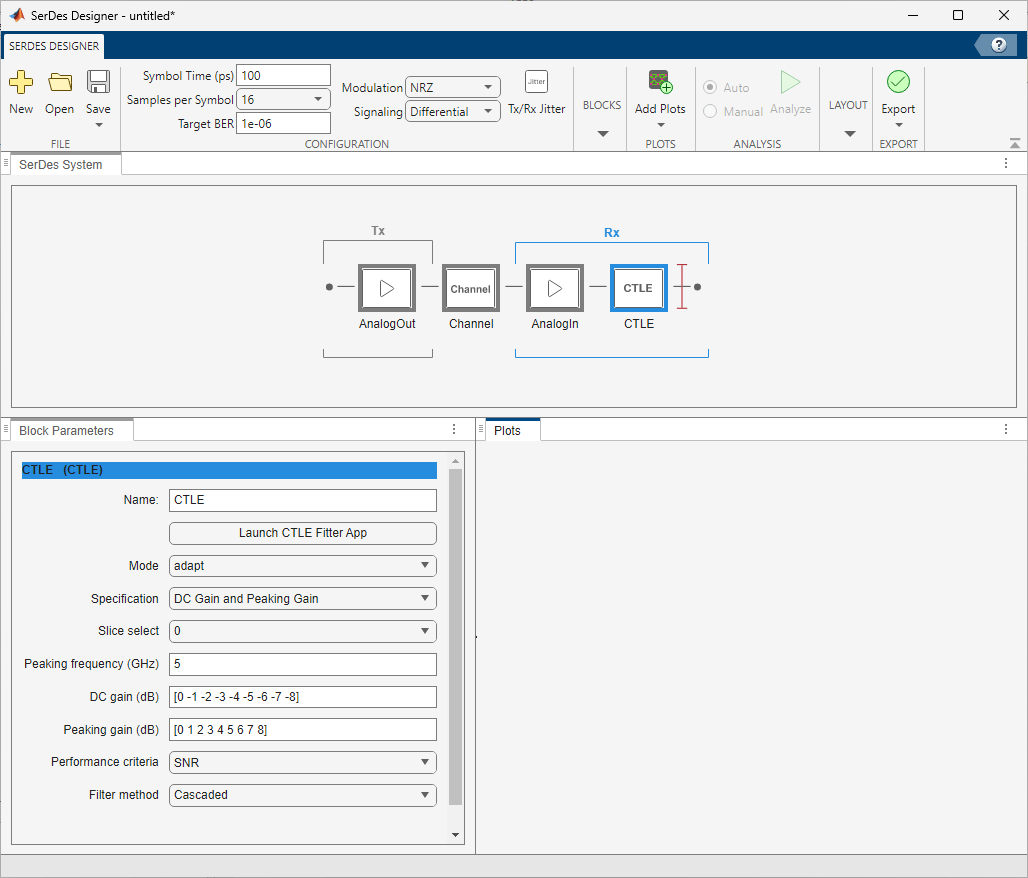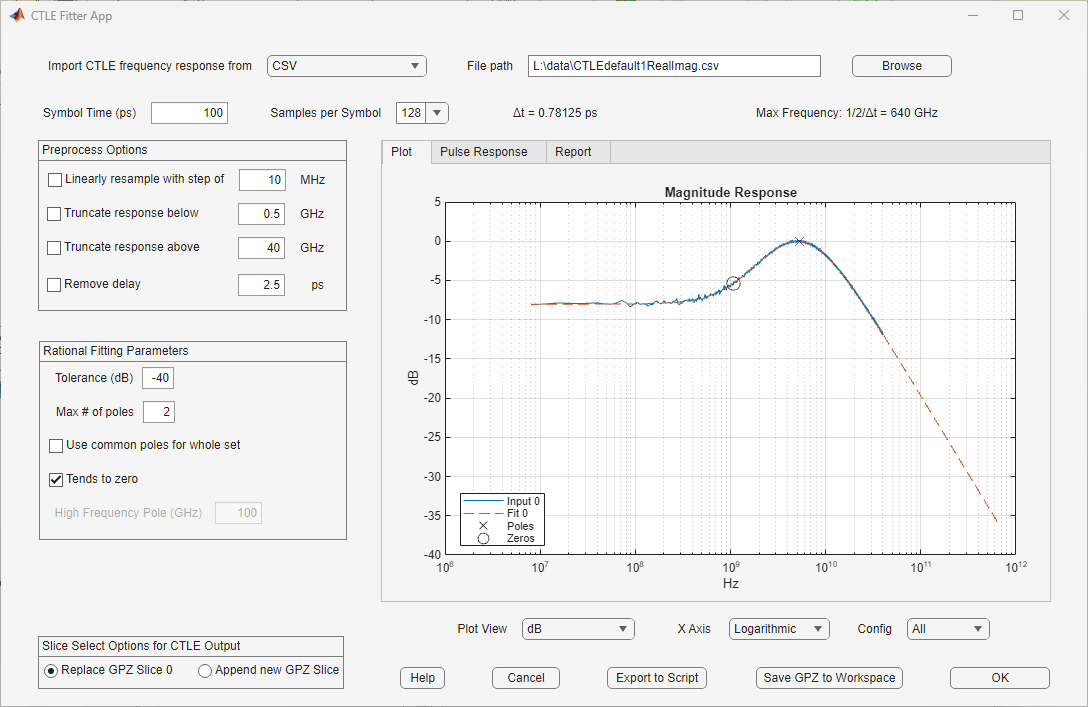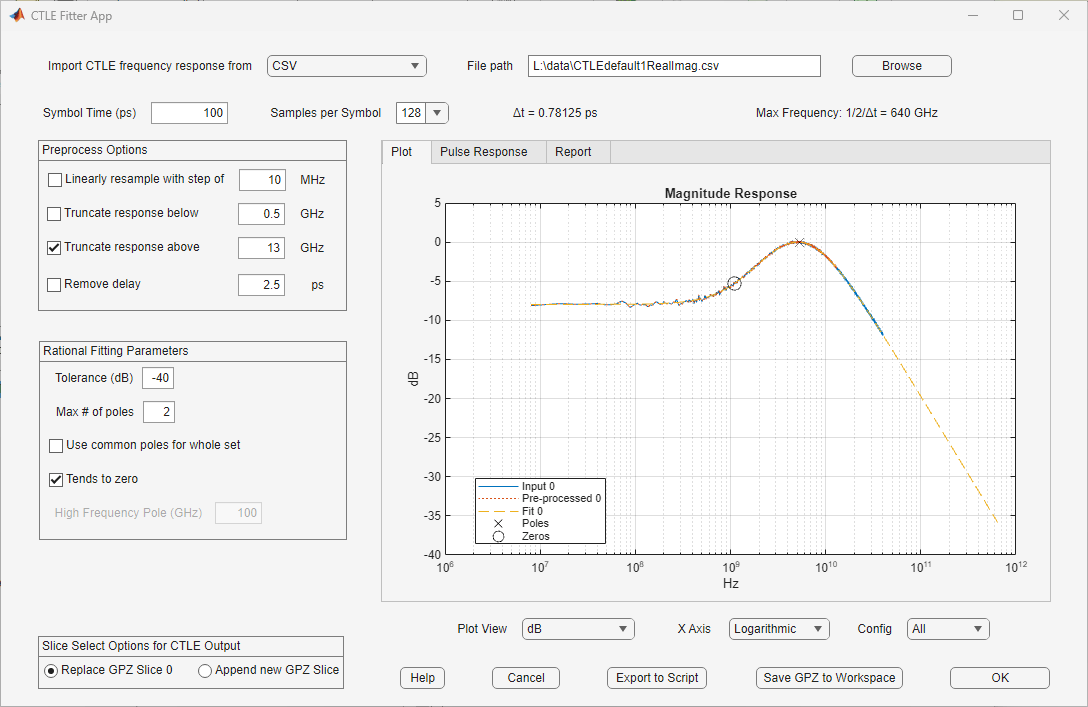# Find Zeros, Poles, and Gains for CTLE from Transfer Function

This example shows how to configure the Specification parameter `GPZ Matrix` of a CTLE in the SerDes Designer app to use zeros, poles, and gains output by the `zpk` function, given poles and residues output by the `rational` function. You can reformat the set of zeros, poles, and gains output by the `zpk` function to use as a `GPZ matrix` in a CTLE block.

### Import Transfer Function

Import a .csv file containing a transfer function using the `readmatrix` function.

```ctle_transfunc = readmatrix('ctle_transfer_function.csv','Range','A7:E775'); rawfreq = ctle_transfunc(:,1); ri = ctle_transfunc(:,4:end);```

### Trim Data to a Specific Cutoff Frequency

As an option, it is possible to truncate the data set from the transfer function used by the Fit. For example you may choose a cutoff frequency of 13 GHz.

```fcutoff = 13e9; ndx = rawfreq<fcutoff;```

### Save Un-truncated Data for Fit Comparison Using Overlay Plot

As an option, the original dataset my be saved for later comparison to the Fit output by the `rational` function.

`rawdata = complex(ri(:,1),ri(:,2));`

### Convert Transfer Function to Complex Form

To prepare data for use by the `rational` function, convert the real numbers from the transfer function to complex numbers using the `complex` function.

```data = complex(ri(ndx,1),ri(ndx,2)); freq = rawfreq(ndx);```

### Find Rational of Transfer Function

You can use the `rational` function to find the best fit to the transfer function. The `rational` function performs iterations to identify a fit with the lowest error. It is important to set the argument `TendsToZero` to `true` to add a pole so that the fit tends to zero as S approaches infinity. This meets the requirement to have one more pole than the number of zeros in the GPZ matrix.

`fit = rational(freq,data,'Tolerance',-40,'TendsToZero',true,'MaxPoles',8,'Display','on');`
```No reduction possible. init: np=0 errdbAAA=0 errdb=0 (np=0) np=0 errdbAAA=-3.33309 errdb=0 (np=0) np=2 errdbAAA=-49.9298 errdb=-50.1853 (np=2) Achieved specified tolerance. final: np=2 errdb=-50.1853 ```

### Convert to Zeros, Poles, Gains from Poles and Residues

The `rational` function returns poles and residues, but you need to convert these into zeros, poles and gains for a CTLE block using function zpk.

`[z,p,~,dcgain]=zpk(fit);`

### Create a GPZ Matrix for CTLE Block from Zeros, Poles, Gains

The zeros, poles and gains output by zpk need to be formatted as a GPZ Matrix for use in a CTLE block. The CTLE can be configured to use Specification parameter `GPZ Matrix` where the units for gains, poles and zeros are dB, Hz, and Hz, respectively. The output of function zpk must be reformatted for these units for use as a `GPZ Matrix.` Note: it is good practice to initialize the `GPZ Matrix` in case the input data set is changed between one analysis to another.

```gpz = zeros(1,length(p)*2); gpz(1,1) = 20*log10(abs(dcgain)); gpz(1,2:2:length(p)*2) = p/(2*pi); gpz(1,3:2:length(z)*2+1) = z/(2*pi); ```

### Plot the Overlay of Fit vs. Data

The `serdes.CTLE` block can be used to generate an overlay plot of the fit results in comparison against the input data set.

```myctle = serdes.CTLE('GPZ',gpz,'SymbolTime',40e-12,'Specification','GPZ Matrix'); [f,H] = plot(myctle); figure(3), semilogx(f*1e-9,db(H),rawfreq*1e-9,db(rawdata)) grid on xlabel('GHz'),ylabel('dB') legend('Fit','Data')```### Configure CTLE Block in SerDes Designer

Launch the SerDes Designer app. Place a CTLE block after the analog model of the receiver. Select the CTLE and from the Block Parameters pane, set the Specification parameter to `GPZ Matrix`. Optionally, you can either type in the name of the `GPZ Matrix` variable (in this example, "`gpz")`, or copy the values from the `GPZ Matrix` cell chart and paste it to the Gain pole zero matrix parameter.### Correlate Pulse Response in SerDes Designer to IBIS-AMI Simulation

In the SerDes Designer app, plot the CTLE Transfer Function and Pulse Response from the Add Plots button. You can move the panes to show two plots by clicking and dragging each pane within the SerDes Designer window.Then click the Export > Make IBIS AMI Model for SerDes System button. The IBIS-AMI model may be loaded into an appropriate EDA tool to plot the Pulse Response from the model. For correlation purposes, you can compare the plots for Pulse Response from the SerDes Designer app and the EDA tool.

| | | (RF Toolbox)

## Support평가판 신청